# Damping with delta-time

Just a quick tip on how to convert usual damping code to something framerate-independent.

Most of us have probably, at some point, written code resembling this:

// Perform velocity damping
velocity -= velocity * 0.01f;


… or probably the more correct:

// Per-second damping coefficient
float const D = 10.0f;
// Damp velocity according to timestep
velocity -= velocity * D * delta_time;


Yet this is not fully framerate-independent; results are slightly different at 30fps and 60fps, and more importantly, spikes in the framerate cause lots of weird artifacts, causing developers to attempt to fix the situation by clamping delta_time, which is not ideal.

## The exponentiation method

Here is one way to fix it: assume that the code works correctly at 60 fps. This means that each frame, velocity is effectively multiplied by 1 - D / 60.

After one second, i.e. 60 frames, velocity has been multiplied by (1 - D / 60) ^ 60.

After two seconds, it has been multiplied by (1 - D / 60) ^ (60 * 2).

After N seconds, it has been multiplied by (1 - D / 60) ^ (60 * N).

So, there, we have a formula that tells us what happens after N seconds, and it’s a continuous function. We can therefore choose N as we like, and especially N = delta_time:

// Per-second damping coefficient
float const D = 10.0f;
// Damp velocity (framerate-independent)
velocity *= pow(1.f - D / 60.f, 60.f * delta_time);


Which can be conveniently rewritten as:

// Per-second damping coefficient
float const D = 10.0f;
// Exponentiation base for velocity damping
float const D2 = pow(1.f - D / 60.f, 60.f);
// Damp velocity (framerate-independent)
velocity *= pow(D2, delta_time);


# The stolen bytes: Visual Studio, virtual methods and data alignment

This article describes a design choice in the C++ ABI of the Visual Studio compiler that I believe should be considered a bug. I propose a trivial workaround at the end.

TL;DR — if the topmost polymorphic class in a hierarchy has members with alignment requirement N where N > sizeof(void *), the Visual Studio compiler may add up to N bytes of useless padding to your objects.

Update: be sure to read the explanation by Jan Gray, who designed the relevant part of the MS C++ ABI some 22 years ago, in the comments section below.

My colleague Benlitz first hit the problem when trying to squeeze memory out of some of our game’s most often instantiated classes. I think it is best illustrated with the following minimal example:

class Foo
{
virtual void Hello() {}
float f;     /* 4 bytes */
};

class Bar
{
virtual void Hello() {}
float f;     /* 4 bytes */
double d;    /* 8 bytes */
};


This is the size of Foo and Bar on various 32-bit platforms:

Linux x86 (gcc) 8 16 no
Linux ARMv9 (gcc) 8 16 no
Win32 (gcc) 8 16 no
Win32 (Visual Studio 2010) 8 24 yes
Xbox 360 (Visual Studio 2010) 8 24 yes
PlayStation 3 (gcc) 8 16 no
PlayStation 3 (SNC) 8 16 no
Mac OS X x86 (gcc) 8 16 no

There is no trick. This is by design. The Visual Studio compiler is literally stealing 8 bytes from us!

## What the fuck is happening?

This is the memory layout of Foo on all observed platforms: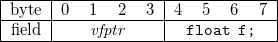The vfptr field is a special pointer to the vtable. The vtable is probably the most widespread compiler-specific way to implement virtual methods. Since all the platforms studied here are 32-bit, this pointer requires 4 bytes. A float requires 4 bytes, too. The total size of the class is therefore 8 bytes.

This is the memory layout of Bar on eg. Linux using GCC: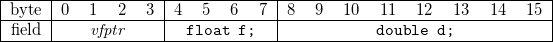The double type has an alignment requirement of 8 bytes, which makes it fit perfectly at byte offset 8.

And finally, this is the memory layout of Bar on Win32 using Visual Studio 2010: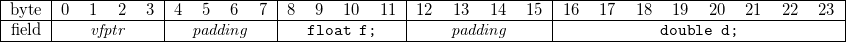This is madness! The requirement for the class to be 8-byte aligned causes the first element of the class to be 8-byte aligned, too! I demand a rational explanation for this design choice.

The problem is that the compiler decides to add the vtable pointer after it has aligned the class data, resulting in excessive realignment.

## Compilers affected

The Visual Studio compilers for Win32, x64 and Xbox 360 all appear to create spurious padding in classes.

Though this article focuses on 32-bit platforms for the sake of simplicity, 64-bit Windows is affected, too.

The problem becomes even worse with larger alignment requirements, for instance with SSE3 or AltiVec types that require 16-byte storage alignment such as _FP128:

class Quux
{
virtual void Hello() {}
float f;     /* 4 bytes */
_FP128 dd;   /* 16 bytes */
};


This is the GCC memory layout on both 32-bit and 64-bit platforms: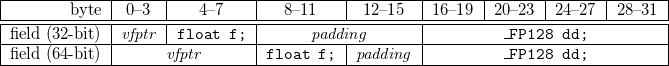The padding there is perfectly normal and expected, because of the alignment requirements for dd.

But this is how Visual Studio decides to lay it out: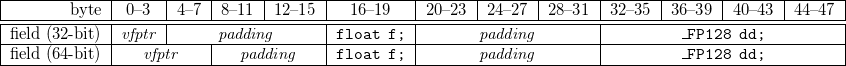That is 16 lost bytes, both on 32-bit and 64-bit versions of Windows.

## Workaround

There is fortunately a workaround if you want to get rid of the useless padding. It is so trivial that it actually makes me angry that the problem exists in the first place.

This will get you your bytes back:

class EmptyBase
{
protected:
virtual ~EmptyBase() {}
};
class Bar : public EmptyBase
{
virtual void Hello() {}
float f;     /* 4 bytes */
double d;    /* 8 bytes */
};


And this is the size of Bar on the same 32-bit platforms:

Platform sizeof(Bar)
Linux x86 (gcc) 16
Linux ARMv9 (gcc) 16
Win32 (gcc) 16
Win32 (Visual Studio 2010) 16
Xbox 360 (Visual Studio 2010) 16
PlayStation 3 (gcc) 16
PlayStation 3 (SNC) 16
Mac OS X x86 (gcc) 16

Phew. Sanity restored.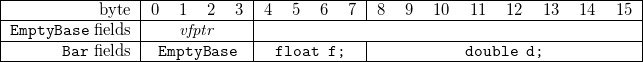The compiler is a lot less confused now: it no longer has to create space for a vfptr in Bar since it is technically already part of EmptyBase.

## Conclusion

Lessons learned:

• The pointer to the vtable isn’t just like any other pointer.
• Various C++ ABIs have different stances on padding and alignment.
• Inheriting from an empty abstract class can make your objects smaller on Windows and Xbox 360!
• Design decisions can haunt you for decades!

The workaround is so simple that it sounds like a good idea to always use it, preemptively.# LINK : fatal error LNK1104: cannot open file ‘XAPID.lib’

Ever got a link error for a library that was referenced nowhere in your Visual Studio project or even in the final link.exe command line? Here's a hint: check the contents of static libraries, too. They may be pulling unexpected dependencies behind your back!

If the static library is part of your solution, here is another hint: check that the [Configuration Properties] >> [C/C++] >> [Code Generation] >> [Runtime Library] configuration values match across projects.

# Beyond De Bruijn: fast binary logarithm of a 10-bit number

Recently I needed a method for retrieving the binary logarithm of a 10-bit number (for the curious, it was for the purpose of converting between 32-bit and 16-bit floating point numbers).

Computing the binary logarithm is equivalent to knowing the position of the highest order set bit. For instance, log2(0x1) is 0 and log2(0x100) is 8.

One well known method for fast binary logarithm is presented at Bit Twiddling Hacks. It is a two-step method where first all lower bits are set to 1 and then a De Bruijn-like sequence is used to perform a table lookup:

int fastlog2(uint32_t v)
{
static const int MultiplyDeBruijnBitPosition =
{
0, 9, 1, 10, 13, 21, 2, 29, 11, 14, 16, 18, 22, 25, 3, 30,
8, 12, 20, 28, 15, 17, 24, 7, 19, 27, 23, 6, 26, 5, 4, 31
};
v |= v >> 1;
v |= v >> 2;
v |= v >> 4;
v |= v >> 8;
v |= v >> 16;
return MultiplyDeBruijnBitPosition[(uint32_t)(v * 0x07C4ACDDU) >> 27];
}


That is 12 integer operations and a table lookup.

## Optimising

It should be obvious what the sequence of operations on v does: fill the integer with ones starting from the highest order bit. Here are a few examples of what happens to v at each step:

 v v |= v >> 1 v |= v >> 2 v |= v >> 4 v |= v >> 8 v |= v >> 16 0x0001 0x0001 0x0001 0x0001 0x0001 0x0001 0x0002 0x0003 0x0003 0x0003 0x0003 0x0003 0x0003 0x0003 0x0003 0x0003 0x0003 0x0003 0x0004 0x0006 0x0007 0x0007 0x0007 0x0007 0x0100 0x0180 0x01e0 0x01fe 0x01ff 0x01ff 0x80000000 0xc0000000 0xf0000000 0xff000000 0xffff0000 0xffffffff

There is one obvious optimisation available: since the input is only 10-bit, the last shift operation v |= v >> 16 can be omitted because the final value was already reached.

int fastlog2(uint32_t v)
{
static const int MultiplyDeBruijnBitPosition =
{
0, 9, 1, 10, 13, 21, 2, 29, 11, 14, 16, 18, 22, 25, 3, 30,
8, 12, 20, 28, 15, 17, 24, 7, 19, 27, 23, 6, 26, 5, 4, 31
};
v |= v >> 1;
v |= v >> 2;
v |= v >> 4;
v |= v >> 8;
return MultiplyDeBruijnBitPosition[(uint32_t)(v * 0x07C4ACDDU) >> 27];
}


10 instructions instead of 12. Not really amazing, but worth mentioning.

## Optimising more?

Could we do better? Now the last line is v |= v >> 8; and it is only useful to propagate the 9th and 10th bits to positions 1 and 2. What happens if we omit that line? Let’s see:

• For most values of v, the expected value is obtained.
• For values of v with a highest order bit at 9th position, 0x1fe could be obtained instead of 0x1ff.
• For values of v with a highest order bit at 10th position, one of 0x3fc, 0x3fd or 0x3fe could be obtained instead of 0x3ff.

The list of possible output values would therefore be 0x1, 0x3, 0x7, 0xf, 0x1f, 0x3f, 0x7f, 0xff, 0x1fe, 0x1ff, 0x3fc, 0x3fd, 0x3fe, 0x3ff. What happens to these values when multiplying them with the De Bruijn sequence? Let's see:

 v v * 0x07C4ACDDU) >> 27 0x1 0 0x3 2 0x7 6 0xf 14 0x1f 30 0x3f 29 0x7f 27 0xff 23 0x1fe 15 0x1ff 16 0x3fc 30 0x3fd 31 0x3fe 0 0x3ff 1

Damn! Two values are colliding. It looks like we cannot omit the last line after all.

## Beyond De Bruijn

Let’s give another try at the problem. Usually De Bruijn sequences are built using either nontrivial algorithms, or brute force. Maybe we could find another sequence that has no collision? Or a sequence that is not a De Bruijn sequence but that works for our problem?

Well, let’s just brute force!

(2 seconds later)

int fastlog2(uint32_t v)
{
static const int MagicTable =
{
0, 1, 2, 8, -1, 3, 5, 9, 9, 7, 4, -1, 6, -1, -1, -1
};
v |= v >> 1;
v |= v >> 2;
v |= v >> 4;
return MagicTable[(uint32_t)(v * 0x5a1a1a2u) >> 28];
}


Down to 8 instructions instead of 12. And the lookup table is now half the size!

## Conclusion

It is possible for multiply-and-shift techniques similar to the De Bruijn sequence algorithm to exist for a larger set of problems. Brute forcing the search is a totally valid method for 32-bit multiplication.

# Maths trick: doing fewer comparisons

Note: this is not an optimisation. It is just one more tool you should have in your toolbox when looking for optimisations. It may be useful.

This is the trick: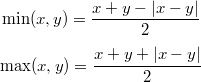You can check for yourself that it is always true: when x > y, |x - y| is the same as x - y, etc.

What good is it for? There is often an implicit comparison in min or max. It might be interesting to replace it with a call to the branchless fabs.

## Example usage

Consider the following code:

float a, b, c, d;
/* ... */
return (a > b) && (c > d);


That kind of code is often used eg. in collision checks, where a lot of tests can be done. This code does two comparisons. On some architectures, this means two branches. Not always something you want.

The test condition is equivalent to:

(a - b > 0) && (c - d > 0)


Now when are two given numbers both positive? That is if and only if the smallest is positive:

min(a - b, c - d) > 0


We may now use our trick:

(a - b) + (c - d) - |(a - b) - (c + d)| > 0


And so the code could be rewritten as such:

float a, b, c, d;
/* ... */
return (a - b) + (c - d) > fabsf((a - b) - (c - d));


We basically replaced the additional test with a call to fabsf and some additions/subtractions. It may be possible to reorganise the input data so that this second version performs better.

# C++ trick: selectively restrict implicit conversions

TL;DR: given a class Foo with an implicit constructor from int, how to allow the implicit conversion in f(42); but not in g(42); where both f and g take a Foo const & argument?

## Background

So I have this real class that performs numeric operations that I want use just like any other C++ numeric type. For instance, I can write the following:

float f = 15, g = 3.5;
int x = f / g;


If I decide that I need double precision, I can write:

double f = 15, g = 3.5;
int x = f / g;


And of course, using my real class for even higher precision:

real f = 15, g = 3.5;
int x = f / g;


I like that. I can just write code as usual, and when I need higher precision, I use real instead of double. It's transparent and convenient.

## Implementation example

Here is a highly simplified example of a real class:

struct real
{
inline real(double d) : m_value(d) {}
inline operator int() const { return (int)m_value; }
/* ... */
long double m_value;
};


It is possible to write real f = 15 because of the implicit constructor. Actually, C++ constructors are always implicit unless specified otherwise.

It is possible to write int x = f / g because of the conversion operator.

So far, so good.

## The problem with implicit promotion

Here is how fabs could be implemented:

real fabs(real const &r)
{
return real(r.m_value < 0 ? -r.m_value : r.m_value);
}


But now we have a problem. A subtle problem. Consider the following code:

double x = fabs(-5.0);


What does this do? Well, it depends. It depends whether <cmath> was included or not. Because if <cmath> wasn’t included, then that code is going to automatically promote -5.0 to a real and call our custom function instead of the one provided by the math library! With no compile-time warning!

This is confusing. It should not happen. But it is a well known problem and there are several obvious workarounds:

1. What most professional C++ programmers will tell you: use namespaces
2. Mark the real(int) constructor explicit

The problem with 1. is that I am not a professional C++ programmer. I am a C programmer who uses C++. I use preprocessor macros and printf and memalign and goto. Try and stop me!

The problem with 2. is that I can no longer write real f = 15, I would need real f(15) or real f = real(15) instead. This is not acceptable, I want real to behave exactly like float and others, to the fullest extent of what the language allows.

## Another solution

Fortunately, the C++ standard has a solution for us: “Implicit conversions will be performed [...] if the parameter type contains no template-parameters that participate in template argument deduction” (ISO/IEC 14882:1998, section 14.8.1.4). You cannot have implicit conversion and template argument deduction at the same time.

It means we just have to make fabs a template function! Which means making real a template class, too.

A quick way to fix real would be:

/* N is unused */
template<int N> struct real_base
{
inline real_base(double d) : m_value(d) {}
inline operator int() const { return (int)m_value; }
/* ... */
long double m_value;
};
typedef real_base<0> real;


The template argument is useless, unfortunately. It will just have to be here, forever. But who knows, you might find a use for it one day.

And to fix fabs:

/* A generic template declaration is needed */
template<int N> real_base<N> fabs(real_base<N> const &r);
/* Here we just add template<> to the previous version */
template<>
real fabs(real const &r)
{
return real(r.m_value < 0 ? -r.m_value : r.m_value);
}


So, what happens with double x = fabs(-5.0); when we forget to include <cmath> now? Well, here is what GCC says:

In function ‘int main()’:
error: no matching function for call to ‘fabs(double)’
note: candidate is:
note: template<int N> real_base<N> fabs(const real_base<N>&)


It seems we’ve successfully managed to avoid the problematic implicit conversion, yet still allow it in places where it was useful!

So what is the rule? It’s simple: where implicit conversion should not be allowed, make the function a specialised template function.

# C/C++ trick: static string hash generation

I am always interested in having the compiler do more things for me, without giving away code clarity or performance for the convenience. Today a colleague linked me to Pope Kim's Compile-Time Hash String Generation article which is a perfect example of the things I like: hidden syntactic sugar that does useful things.

## Inline hash function

The goal: for a given hash function, write something like HASH_STRING("funny_bone") in the code, and have the compiler directly replace it with the result, 0xf1c6fd7f.

The solution: inline the function and hope that the compiler will be clever enough.

#include <string.h>
#define HASH(str) generateHash(str, strlen(str))

inline unsigned int generateHash(const char *string, size_t len)
{
unsigned int hash = 0;
for(size_t i = 0; i < len; ++i)
hash = 65599 * hash + string[i];
return hash ^ (hash >> 16);
}


Unfortunately Pope ran into several very problematic issues:

• requires heavy optimisation flags (/O2 with Visual Studio, -O3 with g++)
• limited to 10-character strings with Visual Studio
• limited to 17-character strings with g++

I could personally reproduce the g++ limitations. I believe they are more related to loop unrolling limits than to the actual string size, but they indeed make the technique unusable in practice.

## Macro-based hash function

If you read my previous article about C/C++ preprocessor LUT generation, you may remember that it used preprocessor tricks to do loop unrolling.

Hence the following implementation:

#include <string.h>
#include <stdint.h>
#include <stdio.h>

#define H1(s,i,x)   (x*65599u+(uint8_t)s[(i)<strlen(s)?strlen(s)-1-(i):strlen(s)])
#define H4(s,i,x)   H1(s,i,H1(s,i+1,H1(s,i+2,H1(s,i+3,x))))
#define H16(s,i,x)  H4(s,i,H4(s,i+4,H4(s,i+8,H4(s,i+12,x))))
#define H64(s,i,x)  H16(s,i,H16(s,i+16,H16(s,i+32,H16(s,i+48,x))))
#define H256(s,i,x) H64(s,i,H64(s,i+64,H64(s,i+128,H64(s,i+192,x))))

#define HASH(s)    ((uint32_t)(H256(s,0,0)^(H256(s,0,0)>>16)))


It has the following properties:

• works in C in addition to C++
• strings are always optimised away by gcc or g++ (but not always the computation itself)
• hash computation is optimised away by gcc or g++ even with -O, -O1 or -Os
• string size limit is 256 characters (probably more than enough for most uses) and can be manually increased or decreased

The following code:

int main(void)
{
printf("%08x\n", HASH("funny_bone"));
printf("%08x\n", HASH("incredibly_large_string_that_gcc_groks_easily"));
}


Is (correctly) optimised to this with gcc -Os:

  ...
movl    $-238617217, %esi movl$.LC0, %edi
xorl    %eax, %eax
call    printf
movl    $-453669173, %esi movl$.LC0, %edi
xorl    %eax, %eax
call    printf
...


I haven't tested it with Visual Studio. Feedback from this compiler would be very appreciated!

# Better function approximations: Taylor vs. Remez

You may have once crossed this particular piece of magic: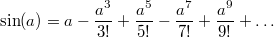The right part is the Taylor series of sin around 0. It converges very quickly to the actual value of sin(a). This allows a computer to compute the sine of a number with arbitrary precision.

And when I say it’s magic, it’s because it is! Some functions, called the entire functions, can be computed everywhere using one single formula! Other functions may require a different formula for different intervals; they are the analytic functions, a superset of the entire functions. In general, Taylor series are an extremely powerful tool to compute the value of a given function with very high accuracy, because for several common functions such as sin, tan or exp the terms of the series are easy to compute and, when implemented on a computer, can even be stored in a table at compile time.

## Approximating sin with Taylor series

This is how one would approximate sin using 7 terms of its Taylor series on the [-π/2, π/2] interval. The more terms, the better the precision, but we’ll stop at 7 for now:

static double taylorsin(double x)
{
static const
double a0 =  1.0,
a1 = -1.666666666666666666666666666666e-1,  /* -1/3! */
a2 =  8.333333333333333333333333333333e-3,  /*  1/5! */
a3 = -1.984126984126984126984126984126e-4,  /* -1/7! */
a4 =  2.755731922398589065255731922398e-6,  /*  1/9! */
a5 = -2.505210838544171877505210838544e-8,  /* -1/11! */
a6 =  1.605904383682161459939237717015e-10; /*  1/13! */
double x2 = x * x;
return x * (a0 + x2 * (a1 + x2 * (a2 + x2
* (a3 + x2 * (a4 + x2 * (a5 + x2 * a6))))));
}


And you may think…“Oh wow that is awesome! So simple for such a difficult function. Also, since I read your masterpiece about polynomial evaluation I know how to improve that function so that it is very fast!”

Well, actually, no.If you are approximating a function over an interval using its Taylor series then either you or the person who taught you is a fucking idiot because a Taylor series approximates a function near a fucking point, not over a fucking interval, and if you don’t understand why it’s important then please read on because that shit is gonna blow your mind.

## Error measurement

Let’s have a look at how much error our approximation introduces. The formula for the absolute error is simple: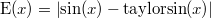And this is how it looks like over our interval:You can see that the error skyrockets near the edges of the [-π/2, π/2] interval.“Well the usual way to fix this is to split the interval in two or more parts, and use a different Taylor series for each interval.”

Oh, really? Well, let’s see the error on [-π/4, π/4] instead:I see no difference! The error is indeed smaller, but again, it becomes extremely large at the edges of the interval. And before you start suggesting reducing the interval even more, here is the error on [-π/8, π/8] now:I hope this makes it clear that:

• the further from the centre of the interval, the larger the error
• the error distribution is very unbalanced
• the maximum error on [-π/2, π/2] is about 6.63e-10

And now I am going to show you why that maximum error value is pathetic.

## A better approximation

Consider this new function:

static double minimaxsin(double x)
{
static const
double a0 =  1.0,
a1 = -1.666666666640169148537065260055e-1,
a2 =  8.333333316490113523036717102793e-3,
a3 = -1.984126600659171392655484413285e-4,
a4 =  2.755690114917374804474016589137e-6,
a5 = -2.502845227292692953118686710787e-8,
a6 =  1.538730635926417598443354215485e-10;
double x2 = x * x;
return x * (a0 + x2 * (a1 + x2 * (a2 + x2
* (a3 + x2 * (a4 + x2 * (a5 + x2 * a6))))));
}


It doesn’t look very different, right? Right. The values a0 to a6 are slightly different, but the rest of the code is strictly the same.

Yet what a difference it makes! Look at this error curve:That new function makes it obvious that:

• the error distribution is better spread over the interval
• the maximum error on [-π/2, π/2] is about 4.96e-14

Check that last figure again. The new maximum error isn’t 10% better, or maybe twice as good. It is more than ten thousand times smaller!!

## The minimax polynomial

The above coefficients describe a minimax polynomial: that is, the polynomial that minimises a given error when approximating a given function. I will not go into the mathematical details, but just remember this: if the function is sufficiently well-suited (as sin, tan, exp etc. are), then the minimax polynomial can be found.

The problem? It’s hard to find. The most popular algorithm to find it is the Remez exchange algorithm, and few people really seem to understand how it works (or there would be a lot less Taylor series). I am not going to explain it right now. Usually you need professional math tools such as Maple or Mathematica if you want to compute a minimax polynomial. The Boost library is a notable exception, though.

But you saw the results, so stop using Taylor series. Spending some time finding the minimax polynomial is definitely worth it. This is why I am working on a Remez framework that I will make public and free for everyone to use, modify and do what the fuck they want. In the meantime, if you have functions to numerically approximate, or Taylor-based implementations that you would like to improve, let me know in the comments! This will be great use cases for me.

# C/C++ trick: static lookup table generation

There are two major ways of using lookup tables (LUTs) in C/C++ code:

• build them at runtime,
• embed them in the code.

One major advantage of runtime initialisation is the choice between static initialisation (at program startup), or lazy initialisation (on demand) to save memory. Also, the generating code can be complex, or use information that is only available at runtime.

In the case of an embedded table, the generation cost is only at compile time, which can be very useful. Also, the compiler may take advantage of its early knowledge of the table contents to optimise code. However, quite often the content of embedded tables is abstruse and hardly useful to someone viewing the code. Usually this is due to the use of an external program for generation, sometimes in a completely different language. But the generation can also often be done using the C/C++ preprocessor.

## Practical example

Consider the bit interleaving routine at Sean Eron Anderson's Bit Twiddling Hacks page (which, by the way, I recommend you read and bookmark). It uses the following LUT (shortened for brevity):

static const unsigned short MortonTable256 =
{
0x0000, 0x0001, 0x0004, 0x0005, 0x0010, 0x0011, 0x0014, 0x0015,
0x0040, 0x0041, 0x0044, 0x0045, 0x0050, 0x0051, 0x0054, 0x0055,
0x0100, 0x0101, 0x0104, 0x0105, 0x0110, 0x0111, 0x0114, 0x0115,
... 32 lines in total ...
0x5540, 0x5541, 0x5544, 0x5545, 0x5550, 0x5551, 0x5554, 0x5555
};


The MortonTable256 table has, as its name suggests, 256 elements. It was pregenerated by some external piece of code which probably looked like this:

for (int i = 0; i < 256; i++)
MortonTable256[i] = (i & 1) | ((i & 2) << 1) | ((i & 4) << 2) | ((i & 8) << 3);


The problem with that external piece of code is that it is external. You cannot write it in this form and have it fill the table at compile time.

If you only take the output of this table, the information on how the table was created is lost. It makes it impractical to build another table that has, for instance, all values shifted one bit left. Even if such a table was created using a modified version of the above code, switching between the two tables would be a hassle unless both versions were kept between preprocessor tests.

## Preprocessor iterator

Here is one way to get the best of both worlds. First, declare the following iterator macros. They can be declared somewhere in a global .h, maybe with more descriptive names:

#define S4(i)    S1((i)),   S1((i)+1),     S1((i)+2),     S1((i)+3)
#define S16(i)   S4((i)),   S4((i)+4),     S4((i)+8),     S4((i)+12)
#define S64(i)   S16((i)),  S16((i)+16),   S16((i)+32),   S16((i)+48)
#define S256(i)  S64((i)),  S64((i)+64),   S64((i)+128),  S64((i)+192)
#define S1024(i) S256((i)), S256((i)+256), S256((i)+512), S256((i)+768)


Their purpose is simple: calling eg. S16(i) will expand to S1(i), S1(i+1), …, S1(i+15). Similarly, S256(i) will call S1 with values from i to i + 255 times.

And this is how to use them in our example:

static const unsigned short MortonTable256 =
{
#define S1(i) ((i & 1) | ((i & 2) << 1) | ((i & 4) << 2) | ((i & 8) << 3))
S256(0)
#undef S1
};


That's it! The table will be built at compile time, and you get to keep the logic behind it.

## A more complex example

Jeroen van der Zijp's fast half float conversions paper describes table-based methods to convert between 16-bit and 32-bit floating point values. The construction of one of the LUTs is as follows:

void generatetables(){
for(unsigned int i=0; i<256; ++i){
int e=i-127;
if(e<-24){                  // Very small numbers map to zero
basetable[i|0x000]=0x0000;
basetable[i|0x100]=0x8000;
} else if(e<-14){             // Small numbers map to denorms
basetable[i|0x000]=(0x0400>>(-e-14));
basetable[i|0x100]=(0x0400>>(-e-14)) | 0x8000;
} else if(e<=15){             // Normal numbers just lose precision
basetable[i|0x000]=((e+15)<<10);
basetable[i|0x100]=((e+15)<<10) | 0x8000;
} else if(e<128){             // Large numbers map to Infinity
basetable[i|0x000]=0x7C00;
basetable[i|0x100]=0xFC00;
} else{                       // Infinity and NaN's stay Infinity and NaN's
basetable[i|0x000]=0x7C00;
basetable[i|0x100]=0xFC00;
}
}
}


And this is the compile-time version :

static uint16_t const basetable =
{
#define S1(i) (((i) < 103) ? 0x0000 : \
((i) < 113) ? 0x0400 >> (0x1f & (113 - (i))) : \
((i) < 143) ? ((i) - 112) << 10 : 0x7c00)
S256(0),
#undef S1
#define S1(i) (0x8000 | basetable[i])
S256(0),
#undef S1
};


In this case the macro code is slightly bigger and was slightly rewritten, but is no more complicated than the original code. Note also the elegant reuse of previous values in the second half of the table.

This trick is certainly not new, but since I have found practical uses for it, I thought you may find it useful, too.

# Understanding basic motion calculations in games: Euler vs. Verlet

During the past month, I have found myself in the position of having to explain the contents of this article to six different persons, either at work or over the Internet. Though there are a lot of articles on the subject, it’s still as if almost everyone gets it wrong. I was still polishing this article when I had the opportunity to explain it a seventh time.

And two days ago a coworker told me the source code of a certain framework disagreed with me… The kind of framework that probably has three NDAs preventing me from even thinking about it.

Well that framework got it wrong, too. So now I’m mad at the entire world for no rational reason other than the ever occurring realisation of the amount of wrong out there, and this article is but a catharsis to deal with my uncontrollable rage.

## A simple example

Imagine a particle with position Pos and velocity Vel affected by acceleration Accel. Let’s say for the moment that the acceleration is constant. This is the case when only gravity is present.

A typical game engine loop will update position with regards to a timestep (often the duration of a frame) using the following method, known as Euler integration:

Particle::Update(float dt)
{
Accel = vec3(0, 0, -9.81); /* Constant acceleration: gravity */
Vel = Vel + Accel * dt;    /* New, timestep-corrected velocity */
Pos = Pos + Vel * dt;      /* New, timestep-corrected position */
}


This comes directly from the definition of acceleration:Putting these two differential equations into Euler integration gives us the above code.

## Measuring accuracy

Typical particle trajectories would look a bit like this:These are three runs of the above simulation with the same initial values.

• once with maximum accuracy,
• once at 60 frames per second,
• once at 30 frames per second.

You can notice the slight inaccuracy in the trajectories.

You may think…“Oh, it could be worse; it’s just the expected inaccuracy with different framerate values.”

Well, no.

Just… no.If you are updating positions this way and you do not have a really good reason for doing so then either you or the person who taught you is a fucking idiot and should not have been allowed to write so-called physics code in the first place and I most certainly hope to humbly bestow enlightenment upon you in the form of a massive cluebat and don’t you dare stop reading this sentence before I’m finished.

## Why this is wrong

When doing kinematics, computing position from acceleration is an integration process. First you integrate acceleration with respect to time to get velocity, then you integrate velocity to get position.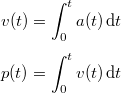The integral of a function can be seen as the area below its curve. So, how do you properly get the integral of our velocity between t and t + dt, ie. the green area below?It’s not by doing new_velocity * dt (left image).

It’s not by doing old_velocity * dt either (middle image).

It’s obviously by doing (old_velocity + new_velocity) * 0.5 * dt (right image).

## And now for the correct code

This is what the update method should look like. It’s called Velocity Verlet integration (not strictly the same as Verlet integration, but with a similar error order) and it always gives the perfect, exact position of the particle in the case of constant acceleration, even with the nastiest framerate you can think of. Even at two frames per second.

Particle::Update(float dt)
{
Accel = vec3(0, 0, -9.81);
vec3 OldVel = Vel;
Vel = Vel + Accel * dt;
Pos = Pos + (OldVel + Vel) * 0.5 * dt;
}


And the resulting trajectories at different framerates:“Oh wow thank you. But what if acceleration is not constant, like in real life?”